# Introduction

JChem Base offers sophisticated chemical formula search for easier usage and better performance. Besides simple chemical formula search, finding isotopes, polymers, multicomponent and non-stoichiometric formulas is also available in JChem Base. The formula search method uses JChem Base's cd_formula column which contains the string formula of the molecule, but it is also possible to use any other custom user column that contain valid chemical formulas.

# Formula search features

The supported chemical formula features are described below.

• Case-insensitive notation of formulas

In case of ambiguous symbols please use the appropriate chemical symbol of the element. Upper case letter defines that a new chemical element is starting. Spaces are ignored between different chemical symbols and numbers both in the query and in the target formula. E.g.: Al2O3 = Al 2 O 3 = Al2 O3
It is useful to separate ambiguous atom symbols with spaces, e.g.: silicon = Si ≠ S I, NaLi = Na Li ≠ N Al I = NAlI.
Accepted forms are e.g.: C7H14O ; c7h14o ; c7H14o ; Si or si for silicon; SI or sI for sulfur and iodine.

• Hill notation is not required

Any order of atoms is accepted, e.g. C7H14O , H14C7O , OC7H14 . A chemical symbol can appear in the formula multiple times, e.g.: CH3COOH = C2H4O2 , both are accepted.

• Parentheses
Parentheses are accepted in case of repeating units and groups. The number after parentheses multiplies each atom in that group. Accepted form is e.g: (C2H4)7O , same as C14H28O .

• Any letter (lower or upper case) after brackets defines a polymer molecule. (C2H4O)n is a polymer molecule with C2H4O repeating unit.

• Combinatorical groups can also be defined by bracketing the conditions. (Br+F+I)5 represents that the sum of bromine, fluorine and iodine atoms in a molecule is 5 (i.e. the molecule contains 4 bromine, 0 fluorine and 1 iodine atom or 1 bromine, 2 fluorine and 2 iodine atoms, etc.). This type of formula presentation is valid only on the query side of formula search.

• Nested parentheses are not supported.

• Defining intervals

Both open and closed intervals are interpreted to set minimum and/or maximum number of type of atoms and groups.
As a formula e.g.: C-7 H10-14 O0- signifies molecules with maximum 7 carbon atoms, minimum 10 and maximum 14 hydrogen atoms and any number of oxygen atoms both on query and target side.
Query form e.g.: (CH2)5-7 O0- N-3 signifies molecules with carbon atoms between 5 and 7, hydrogen atoms between 10 and 14, any number of oxygen atom and maximum 3 nitrogen atoms.

• Multicomponent search

The components should be separated with a period ( . ) in multicomponent search. The sequence of the components is not important. The coefficient of each component can be set by the appropriate numbers, fractions or intervals. As a special index 'x' signs any number of the indicated component in this formula. An omitted coefficient defaults to 1.
Accepted forms are e.g: 5C4H6.Na , 3/4 Na . 2-5 C4H6 , xCuCl2.xH2O means any number of CuCl2 with any number of H2O . (C2H4O.C8H9)n defines a copolymer with C2H4O and C8H9 repeating units.

• Isotopes

Isotope search is available using square brackets in the search formula. Accepted form is [mass number followed by chemical symbol], e.g.: C7H12 [2H][3H] O
Trivial abbreviations ([2H] = D; [3H] = T) are also accepted without square brackets! Example: C7H12 DT O

# Other important aspects

• When no exact number is specified for an atom it is handled as 1, e.g.: C7H14O = 'C7H14O1'.

See more examples of acceptable formulas in JChem Base.

# Search types

To fulfil every requirement, three search types are available to find molecules by chemical formula: Exact , Exact subformula and Subformula search.

Exact search

• The result list contains molecular formulas equal to the given search criteria, atoms with differing numbers and other atom types are not allowed to be present.

Exact subformula search

• The result list contains molecular formulas equal to the given search criteria, atoms with differing numbers will not, but other atom types may be present. E.g. query formula C6 H6 O6 matches C6 H6 O6 S but does not match C4 H6 O6

Subformula search

• The result list contains molecular formulas matching at least the given search criteria but higher number of atoms and other atom types may also be present. E.g. query formula C6 H6 O2 matches C6 H12 O6, C6 H6 O2 S but does not match C4 H6 O6 or C2 H6 O N . According to search rules, in subformula search the query formula can match polymer formula as well (see Table 2.).

Table 1. Search type results comparison

 Query Formula C7 H14 O Result Yes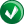No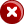Exact C7H14O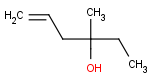C7H14O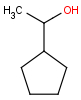C6H14O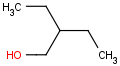C7H14OS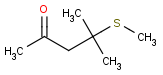Exact Subformula C7H14OSC7H14OSi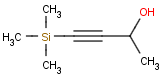C6H14OC7H16OSi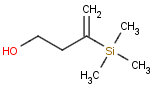Subformula C7H16OSiC8H14O2S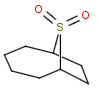C6H14OC7H14S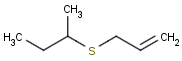Table 2. Query formula can match polymer in Subformula search

 Query Formula C4 H6 O2 Search type Exact Exact subformula Subformula Target formula (C4H8O4)n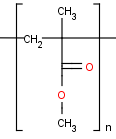(C4H8O4)n(C4H8O4)nFind NoNoYes# Sophisticated Chemical Formula Search Examples

Here you can find some examples on accepted query formulas in JChemBase.

# Formula Examples

 Spaces can divide the formula at any logical point. Even mixed upper case and lower case letters are accepted for chemical symbols. Any order of the elements is accepted. The groups in parentheses are multiplied. Any element can be excluded by zero. C9H21O5PSi C9H21O5PSi c9h21o5psi C9 O5 H21 Si P (CH3)3 (CH2)6 O5 Si P C9 O5 H21 Si P N0 C9 H21 O5 P Si c9 H21 O5 p Si Si P O5 H21 C9 (CH2)9 H3 O5 Si P Si P O5 H21 C9 S0 C 9 H 21 O 5 P Si c 9 h 21 o 5 p si H21 Si O5 P C9 (CH4O)5 C4 H Si P H21 Si O5 P C9 Cl0

# Parentheses usage

 works as a mathematical logic defines polymer molecule specifies combined groups (CH3)3 Si C3H5 (C8H8)n (F+Cl+Br+I)1 Sum of F, Cl, Br and I is equal to 1, i.e. there is only 1 halogen atom in the molecule. C3H9 Si C3H5 (C8H8)L (Cl+Br+I) Sum of Cl, Br and I is equal to 5 in a molecule . C6H14 Si polystyrene (F+Cl+Br+I)0 No halogens are allowed in the molecule.

# Intervals

Open intervals

• 0- : from zero to infinite (none and any)

• 4- : the number of the signed element is greater than or equal to 4.

• -4 : the number of the signed element can be none, 1, 2, 3, or 4.

Closed intervals

• 3-8 : the number of the signed element is greater than or equal to 3 and less than or equal to 8.

For example: (CH2)5-7 O0- N-3 signifies molecules with carbon atoms between 5 and 7, hydrogen atoms between 10 and 14, any number of oxygen atom and maximum 3 nitrogen atoms.

## Interval Example:

 Query formula Results in Exact formula search (CH2)5-7 O0- N-3 C5H10N3 C5H11N3 C5H12N3 C5H13N3 C5H14N3 C6H10N3 C6H11N3 C6H12N3 C6H13N3, ...